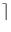Next: Encryption and Decryption with Up: Symmetric Key Cryptography Previous: Secure Byte String (   Contents   Index

# Key for Cryptography ( CryptKey )

Definition

Instances of the class CryptKey store keys for cryptographic algorithms. CryptKey is derived from CryptByteString and hence, its instances also wipe out their memory upon destruction. Apart from the operations of its base class CryptKey provides some static operations which can be helpful for key generation.

#include < LEDA/coding/crypt_key.h >

Creation

 CryptKey k creates an empty key. CryptKey k(uint16 size) creates a key of the given size. All bytes in k are set to zero. CryptKey k(const byte* bytes, uint16 num_key_bytes) initializes k with a copy of the array bytes of size num_bytes. CryptKey k(const CryptByteString& byte_str) initializes k with byte_str. CryptKey k(const char* hex_str) initializes k with the hexadecimal representation in hex_str.

Key generation A key generation algorithm takes as input a (partially) secret seed s of arbitrary length and outputs a key k of the desired size. The gist of this process is to apply a secure hash function H to the seed and to use the returned hash value as the key, i.e. k = H(s). In our implementation we use the checksum of the SHACoder, which is 20 bytes long. Since we want to be able to generate a key of size n! = 20 we use the following approach: We divide the key into p =n/20portions k1,..., kp, where the size of k1,..., kp-1 is 20 and the size of kp is at most 20. We set ki = H(sonoi). (Here ``xoy'' denotes the concatenation of x and y, and kp is possibly a proper suffix of the returned hash value.) Then we have k = k1o...okp.

The question is now what to use as seed for the key generation. The first idea that comes to mind is to use the passphrase supplied by the user. In that case the process would be vulnerable to the so-called dictionary attack. This works as follows: Since many users tend to choose their passphrases carelessly, the attacker builds a dictionary of common phrases and their corresponding keys. This gives him a set of ``likely'' keys which he tries first to break the system. One way to make this attack harder is to apply the hash function several times, i.e. instead of k = H(s) we set k = H(H(...H(s))). Even if we apply H some thousand times it does not slow down the generation of a single key noticably. However, it should slow the generation of a whole dictionary considerably.
An even more effective counter measure is to use something that is called salt. This is a string which is appended to the passphrase to form the actual seed for the key generation. The salt does not have to be kept secret, the important point is that each key generation uses a different salt. So it is impossible to reuse a dictionary built for a specific salt. The salt is also useful if you want to reuse a passphrase. E.g., if you want to authenticate and to encrypt a file you might refrain from remembering two different phrases. But since it is not a good idea to use the same key twice you could generate two different keys by using two different salts.

 CryptKey CryptKey::generate_key(uint16 key_size, const CryptByteString& seed, uint32 num_iterations) generates a key of size key_size by applying a hash function num_iterations times to the given seed. CryptKey CryptKey::generate_key(uint16 key_size, const CryptByteString& passphrase, const CryptByteString& salt = CryptByteString(), uint32 num_iterations = 4096) generates a key from a passphrase and a salt. The seed for the generation is simply passphraseosalt. CryptByteString CryptKey::generate_salt(uint16 salt_size) generates a salt as follows: If salt_size is at least sizeof(date)+4 then a representation of the current date is stored in the last sizeof(date) bytes of the salt. The remaining bytes are filled with pseudo-random numbers from a generator which is initialized with the current time. CryptKey CryptKey::generate_key_and_salt(uint16 key_size, uint16 salt_size, const CryptByteString& passphrase, CryptByteString& salt, uint32 num_iterations = 4096) first some salt is generated (see above); then a key is generated from this salt and the given passphrase. CryptByteString CryptKey::read_passphrase(const string& prompt, uint16 min_length = 6) writes the prompt to stdout and then reads a passphrase from stdin until a phrase with the specified minimum length is entered. (While the phrase is read stdin is put into unbuffered mode.)Next: Encryption and Decryption with Up: Symmetric Key Cryptography Previous: Secure Byte String (   Contents   Index